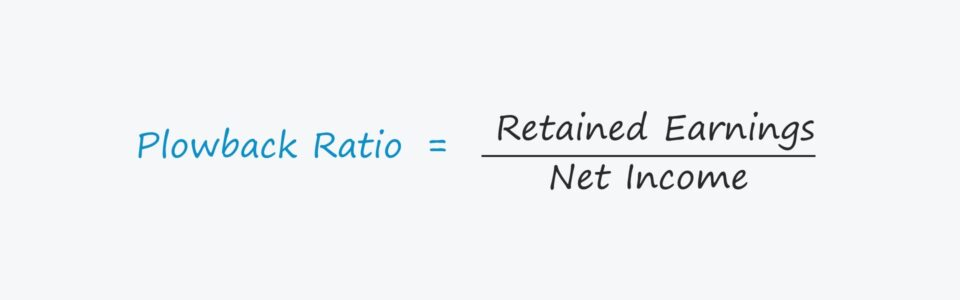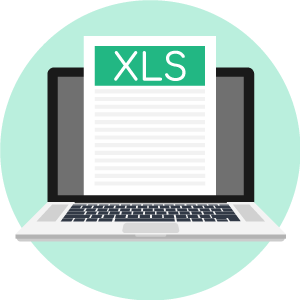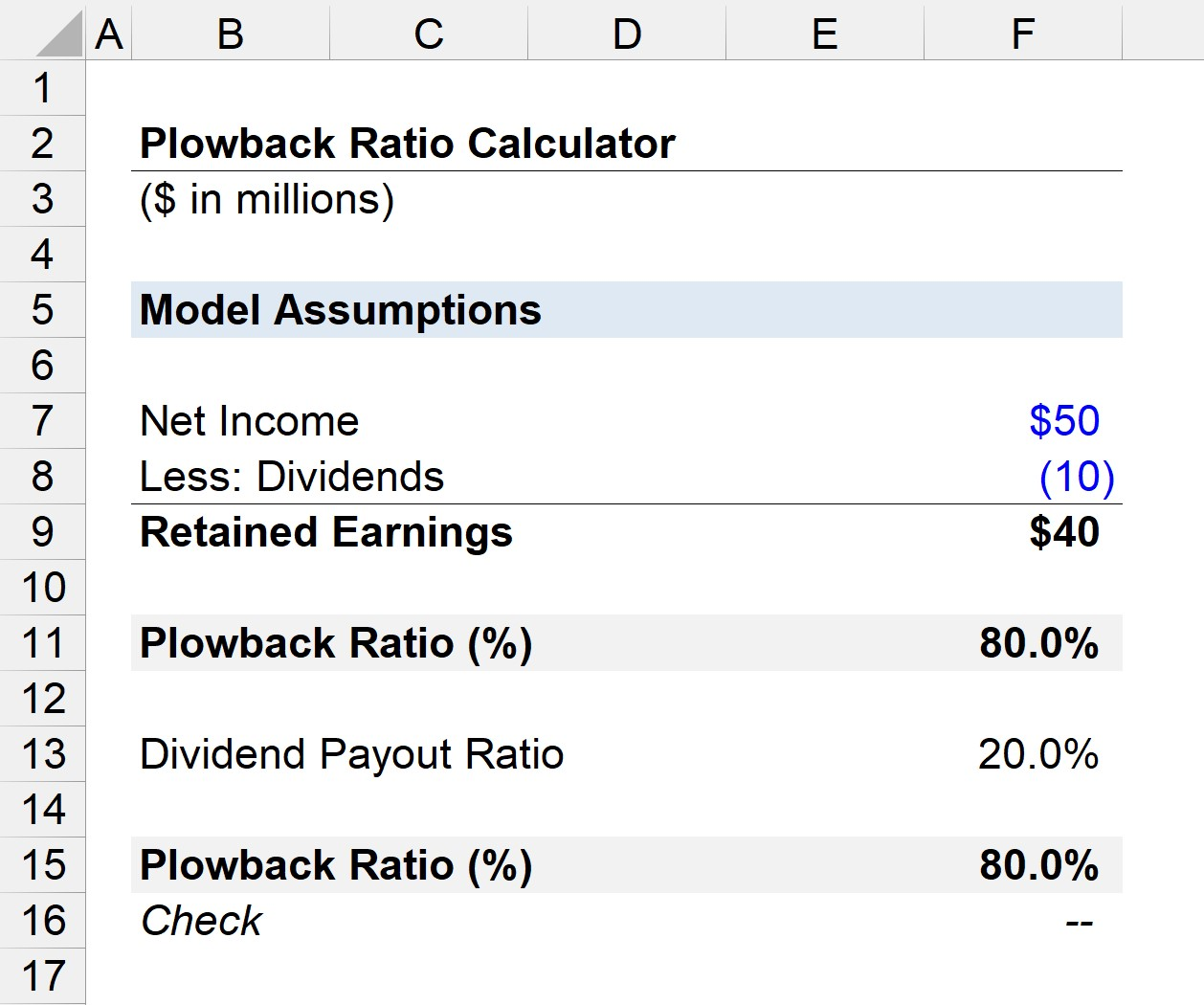# Plowback Ratio

Understand the Plowback Ratio Concept• What is the definition of the plowback ratio?
• What formula calculates the plowback ratio?
• What does the plowback ratio indicate about a company’s growth?
• How is the plowback ratio and the dividend payout ratio related?

## Plowback Ratio Definition

The plowback ratio, also known as the “retention ratio,” is the fraction of a company’s net earnings that are retained to be reinvested into its operations.

Management’s decision to hold onto earnings could suggest that there are currently profitable opportunities worth pursuing.

The inverse of the plowback ratio — the “dividend payout ratio” — is the proportion of net income paid out in the form of dividends to compensate shareholders.

Considering that a higher plowback ratio indicates more growth potential, a higher dividend payout ratio should result in lower growth expectations, i.e. the two are inversely related.

If a company opted to pay out a large percentage of its earnings as dividends, no (or minimal) growth should be expected out of the company.

The rationale behind a long-term dividend program is typically that growth opportunities are limited and the company’s pipeline of potential projects has been exhausted; thus, the best course of action to maximize shareholder wealth is to pay them directly via dividends.

## Plowback Ratio and Implied Growth

A higher plowback ratio implies a higher growth rate, all else being equal.

In theory, greater retention of earnings and rates of reinvestment into profitable projects should coincide with higher near-term growth rates (and vice versa).

As a result, a company’s growth rate (g) can be approximated by multiplying its return on equity (ROE) by its plowback ratio.

###### Growth Formula
• g = ROE × b

Where:

• g = Growth Rate (%)
• ROE = Return on Equity
• b = Plowback Ratio

The plowback ratio, however, cannot be used as a standalone metric, as just because earnings are retained does not mean it is being spent efficiently.

The plowback ratio should therefore be tracked alongside the following return ratios:

## Plowback Ratio and Company Lifecycle

If a company is profitable at the net income line — i.e. “the bottom line” — there are two main options for management to spend those earnings:

1. Re-Invest: The net earnings can be kept and then be used to fund ongoing operations (i.e. working capital needs), or discretionary growth plans (i.e. capital expenditures).
2. Dividends: The net earnings can be used to compensate shareholders; i.e., direct payments can be made to either preferred and/or common shareholders.

The plowback ratio is generally lower for mature companies with established market shares (and large cash reserves).

But for companies in high-growth sectors at risk of disruption and/or a large number of competitors, constant reinvestments are typically necessary, which leads to lower plowback ratios.

###### Capital-Intensive / Cyclical Industries

Note that not all market-leading, established companies have low retention ratios.

For example, companies operating in capital-intensive industries such as automobiles, energy (oil & gas), and industrials must constantly spend significant amounts of money just to maintain their current output.

Capital-intensive industries are also often cyclical in performance, which further creates the need for retaining more cash on hand (i.e. withstand a slowdown in demand or global recession).

## Plowback Ratio Formula

One method to calculate the plowback ratio is to subtract common and preferred dividends from net income, and then divide the difference by net income.

After dividends for the period have been paid out to shareholders, the residual profits are called retained earnings, i.e. net income minus dividend distributions.

###### Plowback Ratio Formula
• Plowback Ratio = Retained Earnings ÷ Net Income

## Plowback Ratio Calculator – Excel Template

We’ll now move to a modeling exercise, which you can access by filling out the form below.Submitting ...

## Plowback Ratio Example Calculation

Suppose a company has reported a net income of \$50 million and paid \$10 million in dividends for the year.

• Plowback Ratio = (\$50 million – \$10 million) ÷ \$50 million
• Plowback Ratio = 80%

In our illustrative scenario, the plowback ratio is 80%, i.e. the company paid out 20% as dividends and the remaining 80% was kept to be reinvested at a later date.

## Plowback Ratio and Payout Ratio Relationship

A different method to calculate the plowback ratio is to subtract the dividend payout ratio from one.

###### Plowback Ratio Formula
• Plowback Ratio = 1 – Payout Ratio

Recall that the plowback ratio is the inverse of the payout ratio, so the formula should be intuitive since the sum of the two ratios must equal one.

Using the same assumptions as in the prior example, we can calculate the plowback ratio by subtracting 1 minus the 20% payout ratio.

• Payout Ratio = \$10 million ÷ \$50 million
• Payout Ratio = 20%

We can then subtract the 20% payout ratio from 1 to calculate a plowback ratio of 80%, which aligns with the previous calculation.

• Plowback Ratio = 1 – 20%
• Plowback Ratio = 80%## Plowback Ratio — Per Share Calculation

The plowback ratio can also be calculated using per share figures, with the two inputs consisting of:

Let’s assume that a company has reported an earnings per share (EPS) of \$4.00 and paid an annual dividend per share (DPS) of \$1.00.

The company’s dividend payout ratio is equal to the earnings per share (EPS) divided by the dividend per share (DPS).

• Payout Ratio = \$1.00 ÷ \$4.00
• Payout Ratio = 25%

Considering 25% of the company’s net earnings were paid out as dividends, the plowback ratio can be calculated by subtracting 25% from 1.

• Plowback Ratio = 1 – 25%
• Plowback Ratio = .75, or 75%

In conclusion, 75% of the company’s net earnings were kept for future reinvestments while 25% was paid out to shareholders as dividends.Step-by-Step Online Course

#### Everything You Need To Master Financial Modeling

Enroll in The Premium Package: Learn Financial Statement Modeling, DCF, M&A, LBO and Comps. The same training program used at top investment banks.Inline FeedbacksLearn Financial Modeling Online

Everything you need to master financial and valuation modeling: 3-Statement Modeling, DCF, Comps, M&A and LBO.

X

The Wall Street Prep Quicklesson Series

7 Free Financial Modeling Lessons

Get instant access to video lessons taught by experienced investment bankers. Learn financial statement modeling, DCF, M&A, LBO, Comps and Excel shortcuts.